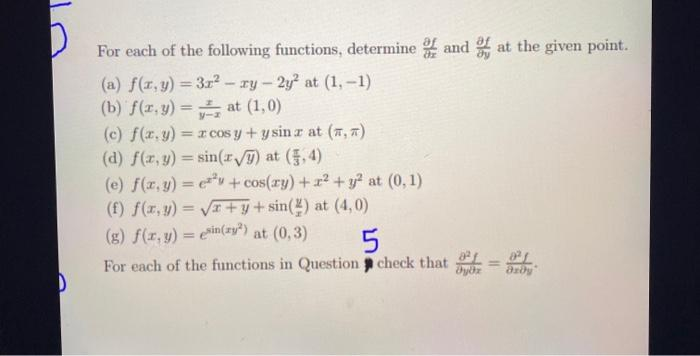### Create an Account

Home / Questions / D For each of the following functions, determine and at the given point. (a) f(x,y) = 3x2 ...

# D For each of the following functions, determine and at the given point. (a) f(x,y) = 3x2 - ry - 2yat (1, -1) (b) f(x,y) = at (1,0) (c) f(x,y) = x cos y + y sin r at (,) (d) f(x,y) = sin(y) at (5, 4)

D For each of the following functions, determine and at the given point. (a) f(x,y) = 3x2 - ry - 2yat (1, -1) (b) f(x,y) = at (1,0) (c) f(x,y) = x cos y + y sin r at (,) (d) f(x,y) = sin(y) at (5, 4) (e) f(x,y) = c*v + cos(xy) + x2 + y at (0,1) (f) f(x, y) = x+y+sin() at (4,0) (g) f(x,y) = exin(xy?) at (0,3) 5 For each of the functions in Question check that DApr 29 2021 View more View LessSubscribe To Get Solution